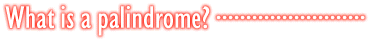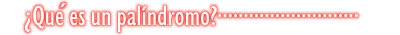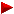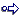HOME plateWON | World!OfNumbersThematic WeblinksPalindromic Records

Introduction

Palindromic numbers are numbers which read the same fromleft to right (forwards) as from the right to left (backwards)Here are a few random examples : 535, 3773, 246191642

Links (old and new) to Webpages dealing with Palindromes

If you meticulously visit and study each one then you'll be more than
introduced into the subject. Have fun! And if you find yourself a source
or reference not listed hereunder please feel free to contact me so that
I can extend and/or update this summary.

References
```

```My site was reviewed and reported on in the october 2004 edition of the NSDL Scout Report for Math, Engineering and Technology.They seek to separate the proverbial 'wheat from the chaff' whenit comes to the innumerable resources available on the web.My site was found to be one of great quality and merit!Integer Sequences from Sloane's OEIS database

1. A006960 Reverse and Add! sequence starting with 196. - N. J. A. Sloane, Simon Plouffe

2. A037076 Palindromes which are the sum of a twin prime pair. - G. L. Honacker, Jr.

3. A045638 Palindromic and divisible by 3. - Jeff Burch
4. A045639 Palindromic and divisible by 4. - Jeff Burch
5. A043040 Numbers that are palindromic and divisible by 5. - Clark Kimberling
6. A045641 Palindromic and divisible by 6. - Jeff Burch
7. A045642 Palindromic and divisible by 7. - Jeff Burch
8. A045643 Palindromic and divisible by 8. - Jeff Burch
9. A045644 Palindromic and divisible by 9. - Jeff Burch

10. A046328 Palindromes with exactly 2 prime factors (counted with multiplicity). - Patrick De Geest
11. A046329 Palindromes with exactly 3 prime factors (counted with multiplicity). - Patrick De Geest
12. A046330 Palindromes with exactly 4 prime factors (counted with multiplicity). - Patrick De Geest
13. A046331 Palindromes with exactly 5 prime factors (counted with multiplicity). - Patrick De Geest
14. A046332 Palindromes with exactly 6 prime factors (counted with multiplicity). - Patrick De Geest
15. A046333 Palindromes with exactly 7 prime factors (counted with multiplicity). - Patrick De Geest
16. A046334 Palindromes with exactly 8 prime factors (counted with multiplicity). - Patrick De Geest
17. A046335 Palindromes with exactly 9 prime factors (counted with multiplicity). - Patrick De Geest
18. A046336 Palindromes with exactly 10 prime factors (counted with multiplicity). - Patrick De Geest
19. A046338 Palindromes with an even number of prime factors (counted with multiplicity). - Patrick De Geest
20. A046341 Palindromes with an odd number of prime factors (counted with multiplicity). - Patrick De Geest
21. A046345 Sum of the prime factors of the palindromic composite numbers (counted with multiplicity). - Patrick De Geest
22. A046348 Palindromes divisible by the sum of their prime factors (counted with multiplicity). - Patrick De Geest
23. A046352 Numbers whose sum of prime factors is palindromic (counted with multiplicity). - Patrick De Geest
24. A046353 Odd numbers whose sum of prime factors is palindromic (counted with multiplicity). - Patrick De Geest
25. A046354 Palindromes whose sum of prime factors is palindromic (counted with multiplicity). - Patrick De Geest
26. A046358 Numbers divisible by the palindromic sum of their prime factors (counted with multiplicity). - Patrick De Geest
27. A046359 Odd numbers divisible by the palindromic sum of their prime factors (counted with multiplicity). - Patrick De Geest
28. A046360 Palindromes divisible by the palindromic sum of their prime factors (counted with multiplicity). - Patrick De Geest
29. A046361 a(n) divided by the palindromic sum of its prime factors is a palindrome (counted with multiplicity). - Patrick De Geest
30. A046362 Composite palindromes divided by the palindromic sum of their prime factors is a palindrome (counted with multiplicity). - Patrick De Geest
31. A046365 Palindromes whose sum of prime factors is prime (counted with multiplicity). - Patrick De Geest

32. A068664 a(1) = 1, a(n) = smallest palindromic multiple of a(n-1). - Amarnath Murthy
33. A068665 a(1) = 3, a(n) = smallest palindromic multiple of a(n-1). - Amarnath Murthy
34. A068666 a(1) = 5, a(n) = smallest palindromic multiple of a(n-1). - Amarnath Murthy
35. A068667 a(1) = 7, a(n) = smallest palindromic multiple of a(n-1). - Amarnath Murthy
36. A068668 a(1) = 9, a(n) = smallest palindromic multiple of a(n-1). - Amarnath Murthy

37. A104444 Not the difference of two palindromes (where 0 is considered a palindrome). - David W. Wilson
38. A108505 The number of palindromic semiprimes less than 10^n. - Robert G. Wilson v

39. A226486 First available increasing palindromes (A002113) found in the decimal expansion of Pi-3 (A000796). - Patrick De Geest & Robert G. Wilson v
40. A226487 First available increasing palindromes (A002113) found in the decimal expansion of the number e-2 (A001113). - Patrick De Geest & Robert G. Wilson v

```

```

[TOP OF PAGE]

Patrick De Geest - Belgium- Short Bio - Some Pictures